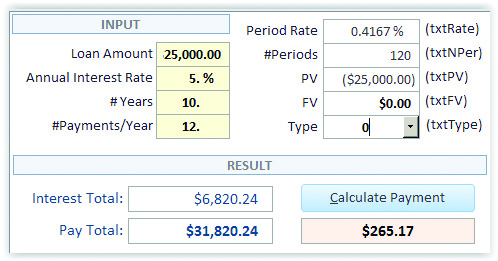Excel has a function called PMT that can be used to calculate the periodic payment for an annuity. We can use it in Access to calculate the monthly payment for a loan. Figure 1 shows a form that calculates the monthly payment based on user-provided information:• The loan amount is \$25,000.
• The annual interest rate is 5%.
• The length of the loan is 10 years.
• The number of payments per year is 12.

See Table 1 for the relevant control names and control sources for the form.With this information, we can use Excel’s PMT function to determine the payment amount shown in the orange control. The PMT function has five arguments:

1. Periodic interest rate (Rate), which is the annual interest rate divided by the number of payments per year;
2. Number of periods (NPer); which is the number of payments in the loan (i.e., the number of years times the number of payments per year).
3. Present value (PV), which, when borrowing, is the negative loan amount;
4. Future value (FV), which is the cash balance you want to attain after the last payment is made (for loans, it would be 0); and
5. Type, which is used to indicate if payments are due at the beginning (value of 0) or end (value of 1) of each period.

The first three are required, and the last two are optional. To be safe, we will specify all five. If the optional arguments don’t have values, we’ll use the same assumptions that Excel uses when they’re left out.

The first step in VBA is to declare an object variable that represents the Excel application. This is to tell Access that it will need to use Excel. There are two ways to declare an object. The first is early binding, which is when the object is declared to be a particular type of object. One reason early binding is more efficient is because Access can check the Excel code when it compiles the rest of the VBA code. An example of early binding is:

Dim oExcel As Excel.Application

This means we plan to use the Microsoft Excel Object library, which includes the PMT function. The problem, however, is that other users may have a different version of Excel. In the Visual Basic Editor, go to Tools, References to see the list of available libraries you can select for early binding. You might see “Microsoft Excel 15.0 Object Library” among the listed libraries. This corresponds to a specific version of Excel (2013 in this case). But someone else might have a different version of Excel, so the 15.0 library might not be correct for one. Early binding would then result in an error.

The other way to declare an object, late binding, doesn’t require that a library be loaded to specify the version of Excel. When code is run, Access determines the version of Excel you have installed and opens the file it needs. Even though late binding is slower, it’s safer and enables the code to be run on more machines without modification. Therefore, we will declare the Excel object variable like this:

Dim oExcel As Object 'Excel.Application

Now that we’ve stated our intention to use Excel, the next statement is what actually creates a new instance of Excel (in essence, it opens Excel) and sets oExcel to use as a reference to it:

Set oExcel = CreateObject("Excel.Application")

Then use Excel to process the PMT worksheet function, pass the five parameters, and return the result to the curPayment variable:

curPayment = oExcel.WorksheetFunction.Pmt _

(Me.txtRate, Me.txtNPer, Me.txtPV, Me.txtFV, Me.txtType)

“Me” indicates the form that the code is behind. Following the period is the name of an object on the form—in this case, a control—and we are getting its value.

Now that we have calculated the amount, the next step is to set the value of the payment textbox control so that it displays the calculated value (e.g., \$265.17) to the user:

Me.txtPayment = curPayment

Finally, to clean up memory being used, quit the Excel instance and release the Excel object variable:

oExcel.Quit

Set oExcel = Nothing

This code is run each time the user clicks the Calculate Payment button, and the result is reported in the appropriate textbox. Download this month’s example database to see the form shown here as well as a different form that contains more calculations and more efficient code that checks to see if Excel is already running and uses that instead of automatically creating a new instance and loading it again. There’s a module with a user-defined function that enables the PMT function to be used by a query. There’s also a query to get the payment for every record of a table with various loan prospects, and a report that groups sets of loans to compare different scenarios.